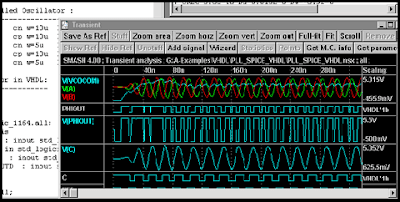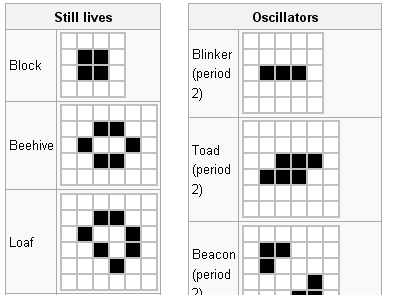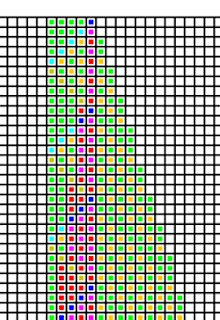## Thursday, February 7, 2013

### Wolfram's Cellular Automata, A New Kind of Science and Example Squaring Rule (2)Overview - Playing the Game Of Life

When most computer users upload a profile image from their desktop to Facebook's website they don't stop to think about the simple binary math rules that are fundamental to most digital devices. We realize that 4 gigabytes of RAM is more memory than 512 megabytes but we don't visualize the logic chips that are involved in an xor \$0x100, eax operation for a 32-bit CISC processor. Software developers have to consider memory management or how a computer's operating system loads their programs into memory. They don't normally consider VHDL logic circuit designs, the data paths, arithmetic logic units or the millions of transistors that make up a modern CPU. Those low-level details have been intentionally hidden from the user application developer. The modern CPU may have changed dramatically over the last decade but at the heart of early digital computing were simple Boolean operations. These simple rules were combined together and logic replicated to load programs into memory and then execute. The rules that control most digital devices are based on elementary Boolean rules. Cellular automata has a similar bottom-up approach, rules consist of simple programs (as Stephen Wolfram calls them) that apply to a set of cells on a grid.Conway's Game of Life cellular automaton is one of the most prominent examples of cellular automata theory. The one dimensional program consists of a cell grid typically with several dozen or more rows and similar number of columns. Each cell on the grid has an on or off Boolean state. Every cell on the grid survives or dies to the next generation depending on the game of life rules. If there are too many neighbors surrounding a cell then the cell dies due to overcrowding. If there is only one neighbor cell, the base cell dies due to under-population. Activity on a particular cell is not interesting but when you run the entire system for many generations, a group of patterns begin to form.

More on Conway's Game of LifeFigure: Game of Life Output

You may notice some common patterns in the figure. After so many iterations through the game of life rules, only a few cells tend to stay alive. We started with a large random number of alive cells and over time those cells died off. In a controlled environment you may begin with carefully placed live cells and monitor the patterns that emerge to model some other natural phenomena.Figure: Common Game of Life Surviving and Oscillating Patterns

A New Kind of Science

The name Stephan Wolfram has been mentioned several times in this post. He is the founder of Wolfram|Research, his company is known for the popular Mathematica software suite and Wolfram|Alpha knowledge engine. He did not initially discover cellular automata but recently he has been a prominent figure in its advocacy. He spent 10 years working on his book, A New Kind of Science. In the 1300 page tome, he discusses how cellular automata can be applied to every field of science from biology to physics. NKA is a detailed study of cellular automata programs.

Basic Cellular AutomataFigure: Wolfram's Elementary CA Rule 30. Look at 3 bit input and 1 bit output.

The diagram above depicts the rule 30 program (or rule 30 elementary cellular automaton). There are 8 input states (2 ^ 3) and an output state of one or zero. If you look at the diagram from left to right. The first sequence of blocks on the left depict an input state of { 1 1 1 } with an output of 0. Given input of cells { 1 1 1}, the output will be set to 0. Subsequently, the next set of blocks consist of an input state of { 1 1 0 } with an output of 0.

Here is python pseudo code for processing rule30 input:
```def rule30(inputCell_0, inputCell_1, inputCell_1): {
if inputCell_0 == 1 and inputCell_1 == 1 and inputCell_2 == 1
return 0:
else if inputCell_0 == 1 and inputCell_1 == 1 and inputCell_2 == 0:
return 0:
...
}
grid = new Grid(100, 100)
grid[row0, col50] = 1 # Enable first cell on row zero
for j until 100:
for i until 100:
valsForNextRow[i] = rule30(inputLastRow[i - 1], inputLastRow[i], inputLastRow[i + 1])```

```Example of first three cases using a boolean notation:
{ 1 1 1 } -> 0
{ 1 1 0 } -> 0
{ 1 0 1 } -> 0
...
Example of first few cases with Scala programming language:

class CellularAutomataRule extends Rule {
def rule(inputState:(Int, Int, Int)) : Rules.Output =
inputState match {
case (1, 1, 1) => 0
case (1, 1, 0) => 0
case (1, 0, 1) => 0
case (1, 0, 0) => 1
...
}
} // End of Rule```

Figure: Scala Example with pattern matchingFigure: Elementary Automata Grid after several iterations, look at image from top to bottom

Cellular Automata and Squaring Application

How do you square two numbers?

With most popular programming languages you could use infix notation providing an input parameter on the left and an input parameter on the right side of some arithmetic function. With Java, you might write the following code:
```int x = 4 * 4;
Output : 16```

The above snippet is valid code used to multiply four times four with a result of sixteen but it does not say much about the native implementation of the multiplication operator. There are many layers involved with that particular function but they aren't visible to the developer. Is the function implemented and optimized by the compiler or implemented by the runtime environment? It is possible that the operating system may cache the result or build an implementation for the arithmetic operation. Ultimately for most basic integer multiplication or addition, those operations are performed at the hardware level. So how then does the hardware do it?

In the figure depicted below is an AND gate and truth table, the gate takes two Boolean input values and returns the output AND operation. If one is entered in input A and zero is entered into input B, then the output C returned by the AND gate is one. An arithmetic logic unit may perform basic Boolean operations or possibly some form of basic arithmetic. An ALU may consist of AND, XOR and other similar simple gates combined to ultimately perform basic arithmetic, increment, decrement or jump operations. (Most of my comments focus on older generation basic circuits, modern circuit design may not use such techniques or basic components)Figure: Boolean AND Gate, InputA, B and Output

If you start from that basic piece of Java code 4 * 4, there are many levels of software and hardware layers that are involved to implement that operation and then return a result.

I wanted to present basic Boolean arithmetic so that you can see how basic rules can lead to more complex patterns and behavior. One two input AND gate will generate a Boolean result. Several million logic circuits may be used to build a complete CPU. You may already be familiar with the Conway's game of life, an initial grid is created with a random number of initial live cells. We can use a simple cellular automata program to square two integers use the rules described in Wolfram's A New Kind of Science. After so many iterations, a common pattern will emerge and that pattern holds the result of N * N. In our squaring example we started with the input number of enabled cells (N = 4) and after so many iterations a pattern emerged that contained the squaring of the input. In many of Wolfram's Elementary rules, a binary sequence is used for input and output. With the general CA squaring rule, an input and output number ranging from 0 to 7 are defined for each cell.

Squaring RuleFigure: Applet Visual Output Grid for Squaring Cellular Automata

```CellularAutomaton[{
{ 0, Blank[], 3} -> 0,
{ Blank[], 2, 3} -> 3,
{ 1, 1, 3 }   -> 4,
{ Blank[], 1, 4} -> 4,
{ Alternatives[1, 2], 3, Blank[]} -> 5,
{ Pattern[\$`p, Alternatives[0, 1]], 4, Blank[]} -> 7 - \$`p,
{ 7, 2, 6} -> 3,
{ 7, Blank[], Blank[]} -> 7,
{ Blank[], 7, Pattern[\$`p, Alternatives[1, 2]]} -> \$`p,
{ Blank[], Pattern[\$`p, Alternatives[5, 6]], Blank[]} -> 7 - \$`p,
{ Alternatives[5, 6], Pattern[\$`p, Alternatives[1, 2]], Blank[]} -> 7 - \$`p,
{ Alternatives[5, 6], 0, 0} -> 1,
{ Blank[], Pattern[\$`p, Alternatives[1, 2]], Blank[]} -> \$`p,
{ Blank[],  Blank[], Blank[]} -> 0}, {
...
Append[Table[1, {\$CellContext`n\$\$}], 3], 0},
Table -> Expression to N
Append -> Table to 3```

Figure: Notebook Source File For Mathematica, General CA Rule for Squaring Automaton

The general rules for the squaring automaton are similar to the rules that were mentioned for the elementary rule30 program. Integer values (range 0 - 7) are used instead of binary inputs and outputs. The initial row and initial number of cells are represented by the input parameter (N = 4 in our example).
`Example Row: 0 0 0 0 0 3 3 3 3 1 0 0 0 0 `

Besides the first row, the initial grid contains all zeros. On the next sequence, the CA rule for squaring is run against each cell on the second row. On the sequence after that, the CA rule is run against the third row and so on until the last row in the grid has been reached. With a 100 x 100 grid, the output pattern will emerge before row 100 is reached.
```class SquaringRule extends Rules.GeneralRule {
def ruleId() = 132
def rule(inputState:Rules.RuleInput) : Rules.Output =
inputState match {
case (0, _, 3) => 0
case (_, 2, 3) => 3
case (1, 1, 3) => 4
case (_, 1, 4) => 4
case (1 | 2, 3, _) => 5
case (0 | 1, 4, _) => 7 - inputState._1
case (7, 2, 6) => 3
case (7, _, _) => 7
case (_, 7, 1 | 2) => inputState._3
case (_, 5 | 6, _) => 7 - inputState._2
case (5 | 6, 1 | 2, _) => 7 - inputState._2
case (5 | 6, 0, 0) => 1
case (_, 1 | 2, _) => inputState._2
case _   => 0
}
} // End of Rule```

Figure: Scala Source for Squaring Rule uses Pattern Matching

Applied Cellular Automata

Cellular automata is often used with data compression, cryptography, artificial intelligence, urban planning, financial market modeling, music generation, and 3D terrain generation. If you are a software engineer, you may have to step back and consider how cellular automata patterns emerge and understand the nature of the dynamic system before looking for a typical software library. CA is not normally seen in everyday applications. Consider this when you look at some random pattern, don't think of the phenomenon as a random sequence of events that cannot be replicated, think of the event in terms of a cellular automaton. Try to imagine the rules that could model that natural behavior. Modeling seemingly random patterns is an area where cellular automata is being widely used. Urban planning departments are integrating geographic information systems (GIS) with cellular automata in an attempt to predict growth in an area of a city.

Summary

The simple squaring example mentioned in this post merely gives you an overview of a basic cellular automata system. Scientists, biologists, computer scientists and software engineers want to find better ways to observe relationships and patterns that occur in our world. Review Stephen Wolfram's A New Kind of Science to give you an idea for what is possible with seemingly simple rules.

Source Code and Applet

1. doingitwrongnotebook/wiki/CelluarAutomataSquaringApplet
2. SVN source repository directory
3. CelluarAutomataApplet - Test of Elementary Rules
4. Game of Life Applet
5. Full Download Applet Examples (keywords: Scala, Rule30, Rule190, GameOfLife, Wolfram Squaring Rule)Figure: Squaring Cellular Automaton Output, Input = 4 (top of grid), Output = 16 (pattern towards the bottom)

Resources
1. http://www.wolframscience.com/
2. http://www.scala-lang.org/ - Scala Programming Language

--- Berlin Brown (2012)

## Tuesday, January 29, 2013

### Internals of the OpenJDK - HashMap

Here is the implementation of HashMap from OpenJDK 6.  It is interesting how simple it truly is.  Essentially HashMap consists of an array called 'table'.  On the 'put' call, we use the hashcode of the key and then call another hash function, then convert that into an index into the array.  Place the 'value' object at the index array position.OpenJDK HashMap Implementation